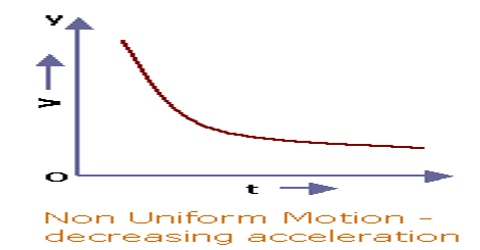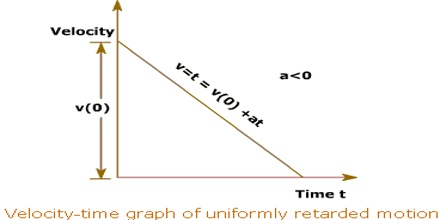Physics

# Retardation or Negative AccelerationRetardation:

The rate of change of velocity with the time of a moving body can either be positive or negative. If the rate of change of velocity with time is positive, it is called acceleration and if negative, it is called retardation. So, when the acceleration is acting in a direction opposite to the velocity, it is called retardation. It is a synonym of the negative acceleration. As acceleration is a vector quantity so it is decided by the coordinate system or reference frame that you use to describe the motion. If it is positive it is termed as acceleration if negative then it is termed as retardation. For example, when you apply a break of your cycle while going to school/college the effect produced by break on Tyre is called retardation.

That means negative acceleration is called retardation.Retardation is measured as an amount by which the velocity of a body changes in one second. The unit and dimension art retardation are the same as that of acceleration. If the velocity of a body is decreasing, then its acceleration will be negative. Negative acceleration is negative but there is no decrease or increase in speed just in case of free fall it is a constant acceleration. The negative acceleration is also called retardation or deceleration.

Example: A train is said to be retarded when it slows down on reaching a station, i.e. the velocity decreases. Retardation is acceleration with a negative sign. Or the negative value of acceleration shows that the velocity of a body is decreasing.

The velocity of a body may increase or decrease. Acceleration takes place when the velocity of a body changes. So when the velocity of a body increase, the acceleration is said to be positive, and when the velocity of a body decrease, the acceleration is said to be negative (Retardation). A body is said to be retarded if its velocity is decreasing.

Example: When something thrown upward the acceleration due to gravity acts downward and taken as negative. When applying a break to stop cycle then also acceleration is negative.